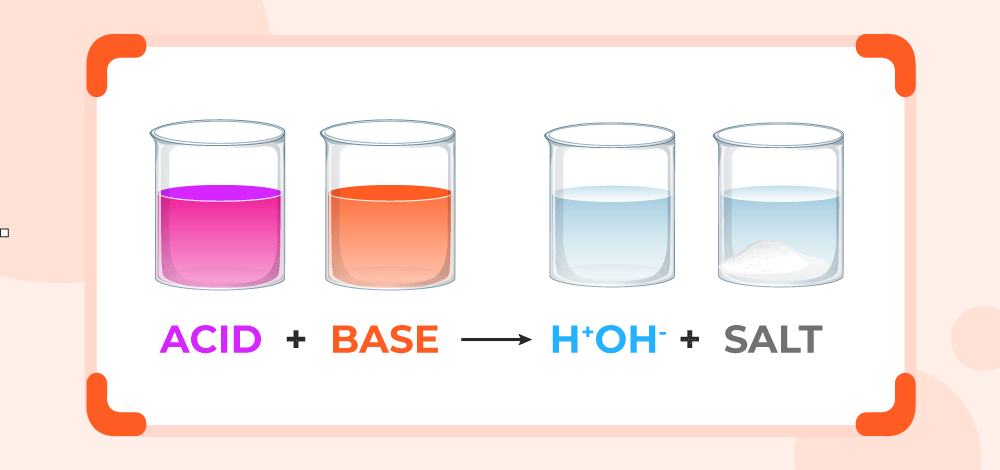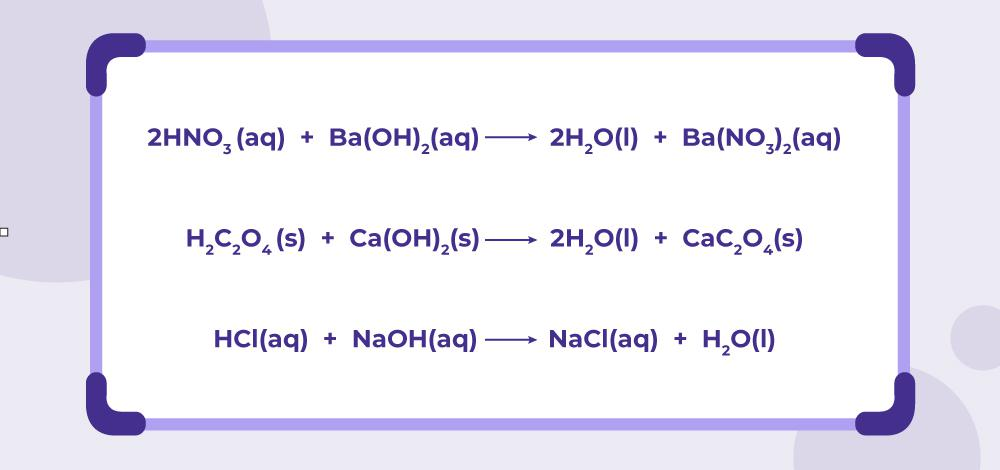GeeksforGeeks App
Open AppBrowser
Continue

## Related Articles

Neutralization Reaction, in chemistry, is a reaction between acid and alkali to produce salt and water. The natural world exhibits pairs that complement and sometimes neutralize each other. For instance, if there is poison, there is also an antidote, and if there is fire, there is also water, which can be regarded as its polar opposite that can neutralize the effect of one another. Life and death are other examples of this complementary pairing. In chemistry, a complementary pair known as Acid-Base exists, which neutralizes each other’s effects and behaves oppositely when treated with various common substances.

## What is Neutralization?

Neutralization is the term used for the reaction between acid and alkali. It is the process in which acid reacts with base or alkali and forms water and salt involving the hydrogen ions (H+) and hydroxide ions (OH) in the process of formation of water molecules. This reaction is a double displacement reaction, where generally radical ions from acid and metal ions from alkali are combined together to form a salt.## Neutralization Reaction: Examples

All the examples generally follow the same reaction pattern for the Neutralization Reaction .i.e, acid and base results in salt and water.## Types of Neutralization Reaction

There are 4 types of Neutralization reaction which depends on the strength of the acid and base taken, which are as follows:

• Strong Acid -Strong Base Neutralization Reaction
• Strong Acid -Weak Base Neutralization Reaction
• Weak Acid -Strong Base Neutralization Reaction
• Weak Acid -Weak Base Neutralization Reaction

### Strong Acid and Strong Base

When a strong acid, such as hydrochloric acid (HCl), reacts with a strong base, such as sodium hydroxide (NaOH), a neutralization reaction occurs. The H+ ions from the acid combine with the OH- ions from the base to form water (H2O) molecules. The remaining ions, Na+ and Cl-, combine to form sodium chloride (NaCl), a salt. The balanced chemical equation for the reaction is:

HCl + NaOH → NaCl + H2O

This reaction releases a large amount of heat and is exothermic. The resulting solution is neutral with a pH of 7.0, as the acidic and basic properties have been cancelled out by the reaction.

### Strong Acid and Weak Base

When a strong acid reacts with a weak base, the resulting salt will be acidic in nature. The reaction between a strong acid and a weak base can be represented by the general equation:

HA + BOH → BA + H2O

In this equation, HA represents a strong acid, BOH represents a weak base, BA represents the salt produced, and H2O represents water.

Overall, the reaction between a strong acid and a weak base will produce a salt that is acidic in nature.

For example, when hydrochloric acid (HCl) reacts with ammonia (NH3), which is a weak base, the following reaction occurs:

HCl + NH3 → NH4Cl

In this reaction, ammonium chloride (NH4Cl) is produced as the salt, and water is not produced.

### Weak Acid and Strong Base

When a weak acid reacts with a strong base, it results in the formation of salt and water.

The general chemical equation for the reaction between a weak acid and a strong base can be represented as:

HA + NaOH → NaA + H2O

where HA is a weak acid and NaOH is a strong base. NaA is the salt formed as a result of the reaction and H2O represents water.

Overall, the reaction between a weak acid and a strong base produces salt and water, and the pH of the resulting solution will be slightly basic due to the presence of excess hydroxide ions (OH).

### Weak Acid and Weak Base

When a weak acid reacts with a weak base, the resulting solution can be either acidic, basic, or neutral, depending on the relative strengths of the acid and base involved. The reaction between a weak acid and a weak base is known as a weak acid-weak base equilibrium reaction.

An example of a weak acid-weak base reaction is the reaction between acetic acid (CH3COOH) and ammonia (NH3). Both acetic acid and ammonia are weak acids and weak bases, respectively. The balanced chemical equation for the reaction is:

CH3COOH + NH3 ⇌ NH4+ + CH3COO

In this reaction, acetic acid donates a proton (H+) to ammonia, which acts as a base and accepts the proton to form an ammonium ion (NH4+). At the same time, the acetate ion (CH3COO) is formed. The reaction is reversible and proceeds in both directions, with the forward reaction favouring the formation of products in a basic medium, whereas the reverse reaction favours the formation of reactants in an acidic medium.

Where Ka and Kb are rate constants of the acid and base reaction respectively.

## Application of Neutralization

Some of the common applications of the Neutralization reaction are as follows:

### Agriculture

Neutralization is a crucial process in agriculture, where acidic soil is neutralized by the use of alkali-based fertilizers to create an ideal condition for crop growth. Wood ash is a commonly used fertilizer in rural areas because it is easily accessible to local farmers.

### Antacid tablets

Antacid tablets leverage the same principle of neutralization to cure the discomfort caused by acidic food during digestion. The stomach uses hydrochloric acid to break down food into simpler molecules that can be easily absorbed by the intestines. To neutralize the excess acid, antacid tablets are formulated with alkalis like aluminium hydroxide, magnesium carbonate, and magnesium trisilicate.

### Bee Sting

A bee sting is also treated with an application of baking soda, as bee sting contains formic acid which can be neutralized by rubbing any basic substance on the bee stung area. Unlike bee stings, a wasp sting is basic in nature, so it can be treated with any acidic substance such as vinegar or any citrus fruit.

### Titration

To find out the concentration of any given acid or base using the titration method, where using acid we determine the unknown strength of the base, and using alkali, we determine the unknown strength of the acid. In chemical labs. This is a very important method to find the unknown strengths of acid or base. If the strength of acid and base is known, we can find the amount of acid required to neutralize alkali or vice versa using the following formula:

Moles of Acid  = Moles of Base

or

Volume of acid × Concentration of H+ ions = Volume of Alkali × Concentration of OH ions

## Solved Examples on Neutralization Reaction

Example 1: How much NaOH of strength 0.5M, is required to neutralize 45 ml of 0.25M HCl?

Solution:

To neutralize 45 ml of 0.25M HCl, we need an equal amount of NaOH of known strength.

and we know for complete neutralization, Moles of HCl = Moles of NaOH

So, Moles of HCl = concentration × volume

⇒ Moles of HCl = 0.25 mol/L × 0.045 L

⇒ Moles of HCl = 0.01125 moles

HCl + NaOH → NaCl + H2O

Since HCl and NaOH react in a 1:1 molar ratio, we need 0.01125 moles of NaOH to neutralize the HCl.

Moles of NaOH = Moles of HCl = 0.01125 moles

Thus, Volume of NaOH = moles of NaOH / concentration

⇒ Volume of NaOH  = 0.01125 moles / 0.5 mol/L

⇒ Volume of NaOH = 0.0225 L = 22.5 mL

Therefore, we need 22.5 mL of 0.5M NaOH to neutralize 45 mL of 0.25M HCl.

Example 2: Consider the titration of 25.00 mL of 0.1 M HBr with 0.2 M KOH. Calculate the volume of  KOH required to neutralize HBr.

Solution:

The balanced chemical equation for the reaction between HBr and KOH is:

HBr + KOH → KBr + H2O

Volume of HBr = 25.00 mL = 0.02500 L

Concentration of HBr = 0.100 M

As, Moles = concentration x volume

Thus, Moles of HBr = 0.100 M x 0.02500 L = 0.00250 mol

Since molar ratio of HBr to KOH in the reaction is 1:1 , the amount of moles of KOH required to neutralize HBr is also 0.00250 mol.

Volume of KOH = Moles / Concentration

⇒ Volume of KOH = 0.00250 mol / 0.200 M

⇒ Volume of KOH = 0.0125 L = 12.5 mL

Therefore, 12.5 mL of 0.2 M KOH is required to neutralize 25.00 mL of 0.1 M HBr.

## FAQs on Neutralization Reaction

### Question 1: What is the Neutralization Reaction?

Chemical reaction involving acid and base as reactant to form salt and water as product, is known as Nuetralization reaction. For example, reaction of hydrochloric acid with sodium hydroxide forms common salt and water i.e.,

HCl + NaOH → NaCl + H2O

### Question 2: What is the heat of neutralization?

Neutralization is a exothermic reaction, as there is heat produced during the reaction. This heat produced during the reaction is called heat of neutralization.

### Question 3: What neutralizes stomach acid?

Antacid tablets which contains some common alkali such as  aluminum hydroxide, magnesium carbonate, and magnesium trisilicate, used to nutralize acidity of the stomach.

### Question 4: Write two examples of Neutralization Reaction.

Reaction of hydrocholoric acid and ammonia,

HCl + NH3 → NH4Cl

Reaction of Sodium Hydroxide and Hrdrocholoric Acid,

HCl + NaOH → NaCl + H2O

are two examples of Nuetralization Reaction.